##### Comparison Operators in PostgreSQL

In PostgreSQL, comparison operators are used for comparing values and determining whether they meet certain conditions/criteria. Postgres offers a wide range of basic and advanced comparison operators to compare values logically. For instance, you can determine if a value is equal to, less than, or greater than, check the existence of some value, etc.

This post will explain the usage of some basic and advanced comparison operators in Postgres via practical examples. So, let’s start!

Comparison Operators in PostgreSQL

Postgres offers various comparison operators, for example, =, <, !=, <=, etc. Postgres also provides advanced comparison operators, such as the BETWEEN operator to check if a value is within a range, the IN operator to check if a value belongs to a list of values, and so on. Postgres offers the following comparison operators:

- “=”: The “equal” operator in Postgres is used to check the equality.
- “!=”: The “not equal” operator opposes the working of the equal operator.
- “<>”: Works the same way as “!=”.
- “>”: The “greater than” operator is used for finding the greater/maximum value.
- “>=”: Finds a value greater than or equal to the specified/given value.
- “<”: Finds the less/minimum value.
- “<=”: Finds a value less than or equal to the given/specified value.
- BETWEEN: Finds a value within the specified range.
- LIKE: Performs pattern matching.
- IS NULL: Checks a null value.
- IS NOT NULL: Checks a non-null value.
- IN: Check if a value belongs to a list of values.
- NOT: Retrieves the opposite results for the specified condition.

Let's put these operators into practice and see how they work.

Example 1: How to Use = Operator in Postgres?

We have already created a table named “emp_information”, whose data is shown in the following snippet:

`SELECT * FROM emp_information;`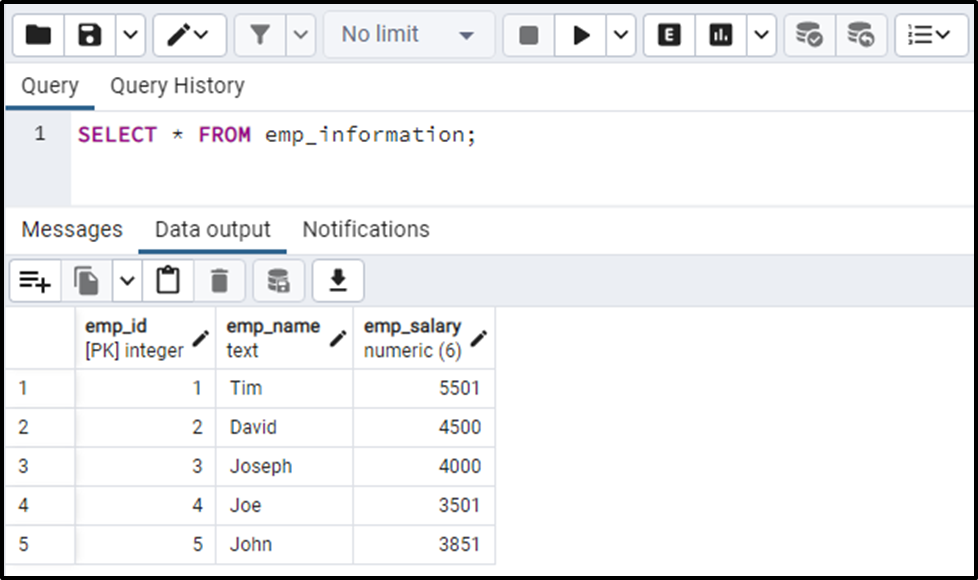Suppose we want to find the name of an employee whose salary equals “4000”. For that, we will use the “=” operator as follows:

```SELECT emp_name
FROM emp_information
WHERE emp_salary = 4000;```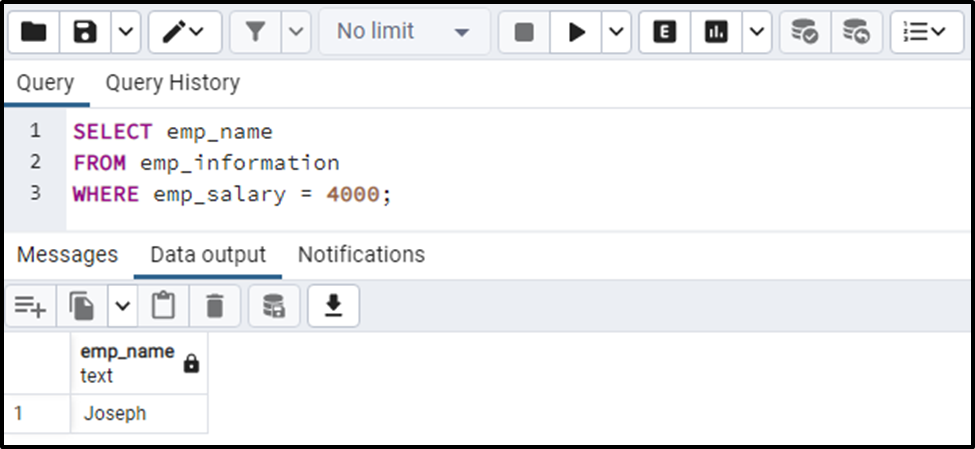The output proves the working of the equal “=” operator.

Example 2: How to Use <> Operator in Postgres?

In this example, we will use the not equal to operator to fetch those employees whose salary is not equal to “4000”:

```SELECT emp_name, emp_salary
FROM emp_information
WHERE emp_salary <> 4000;```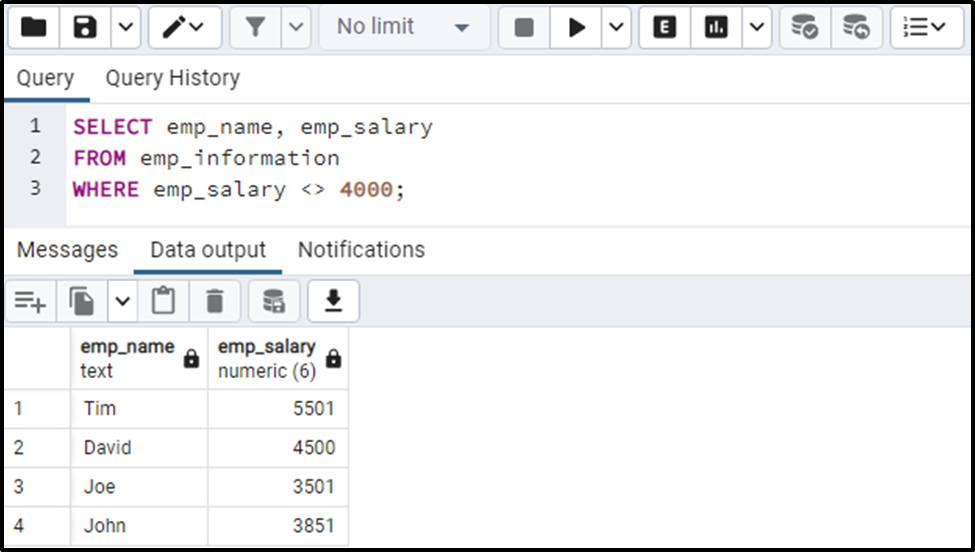The output proves the working of the not equal operator in Postgres.

Example 3: How to Use Greater Than > Operator in Postgres?

Let’s learn how the greater-than operator works in Postgres:

```SELECT emp_name, emp_salary
FROM emp_information
WHERE emp_salary > 4000;```

The above query will retrieve the names of the employees whose salary is greater than “4000”: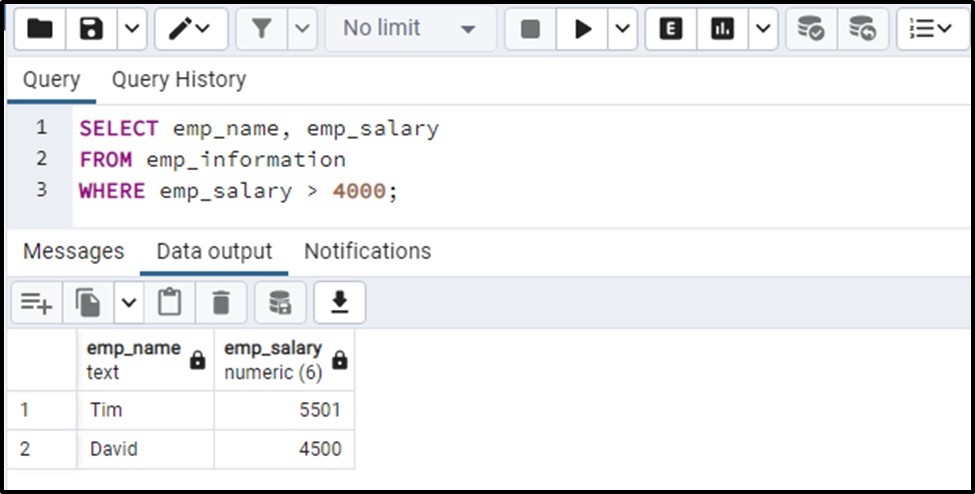The output proves the working of the greater than “>” operator.

Example 4: How to Use Less Than or Equal to <= Operator in Postgres?

This example will show you the working of the “<=” operator:

```SELECT emp_name, emp_salary
FROM emp_information
WHERE emp_salary <= 4000;```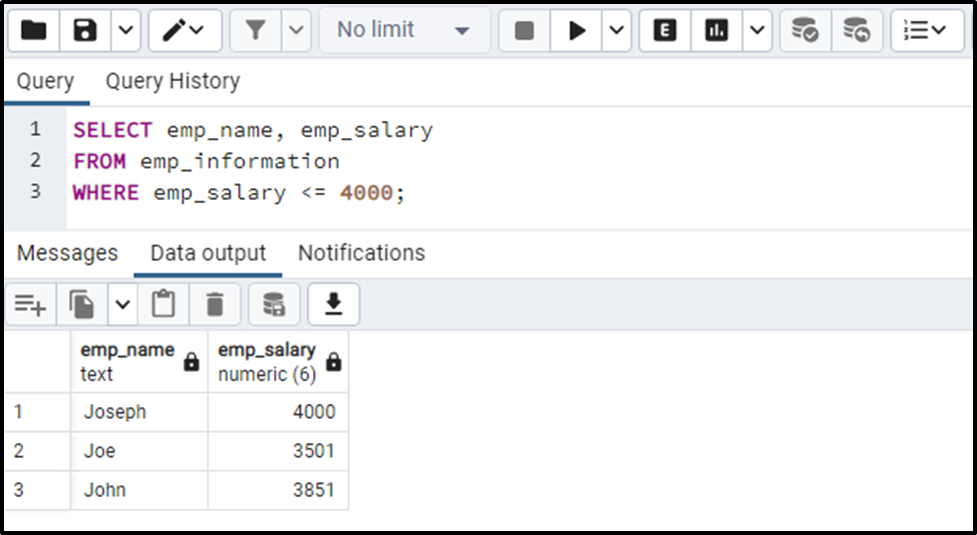This way, the “less than or equal to” operator works in Postgres.

Example 5: How to Use Between Operator in Postgres?

Suppose we want to fetch all those employees whose salary is greater than “3800” but less than “5000”. For this purpose, we will use the BETWEEN operator as follows:

```SELECT emp_name, emp_salary
FROM emp_information
WHERE emp_salary BETWEEN 3800 AND 5000;```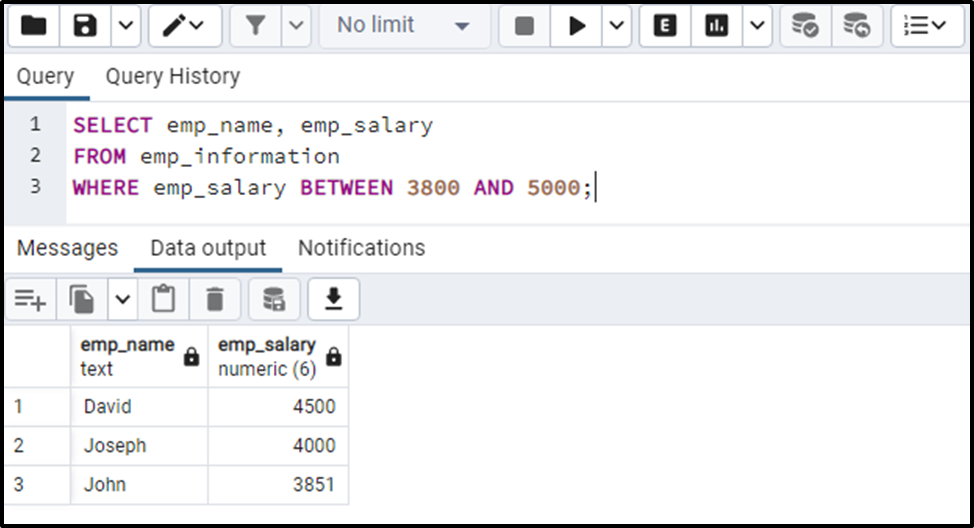The output proves the working of the BETWEEN operator.

Example 6: How to Use NOT Operator in Postgres?

Using the NOT operator with BETWEEN operator will negate the original result of the BETWEEN operator:

```SELECT emp_name, emp_salary
FROM emp_information
WHERE emp_salary NOT BETWEEN 3800 AND 5000;```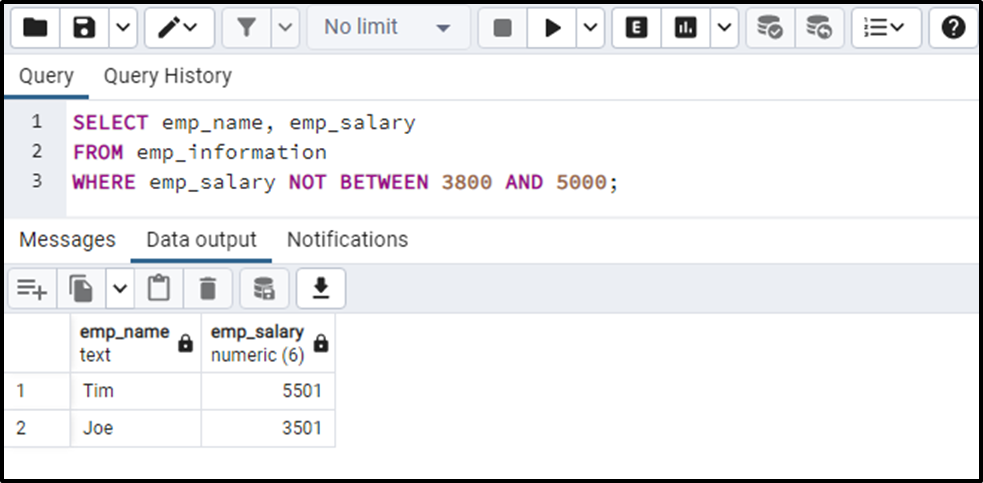The above snippet proves the working of the NOT operator in Postgres.

Example 7: How to Use IN Operator in Postgres?

Let’s learn how to use the IN operator in Postgres via the following example:

```SELECT emp_name, emp_salary
FROM emp_information
WHERE emp_salary IN(3800, 3851, 4500, 5000);```

The IN Operator will check the existence of the specified values in the emp_salary column: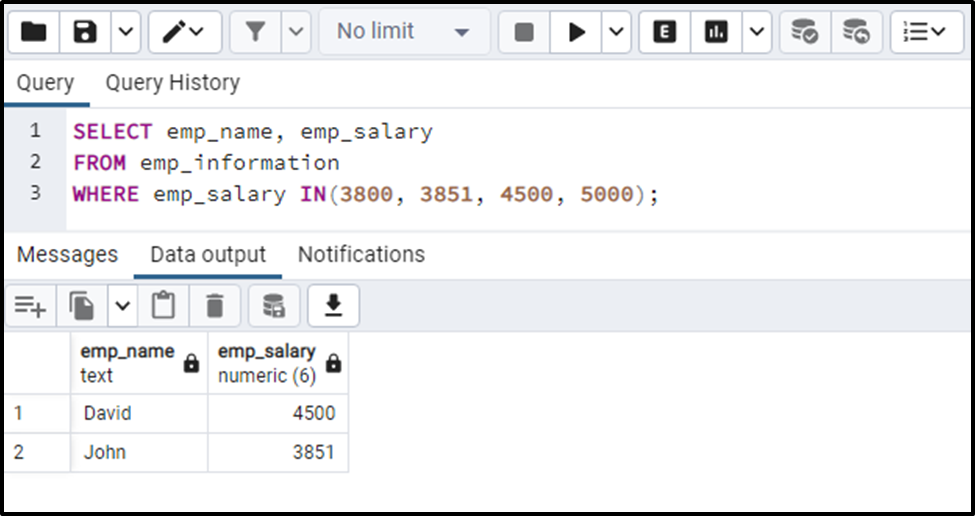The output snippet authenticates the working of the IN operator.

Example 8: How to Use IS NOT NULL Operator in Postgres?

The below-specified query will retrieve the non-null values from the “emp_salary” column:

```SELECT emp_name, emp_salary
FROM emp_information
WHERE emp_salary IS NOT NULL;```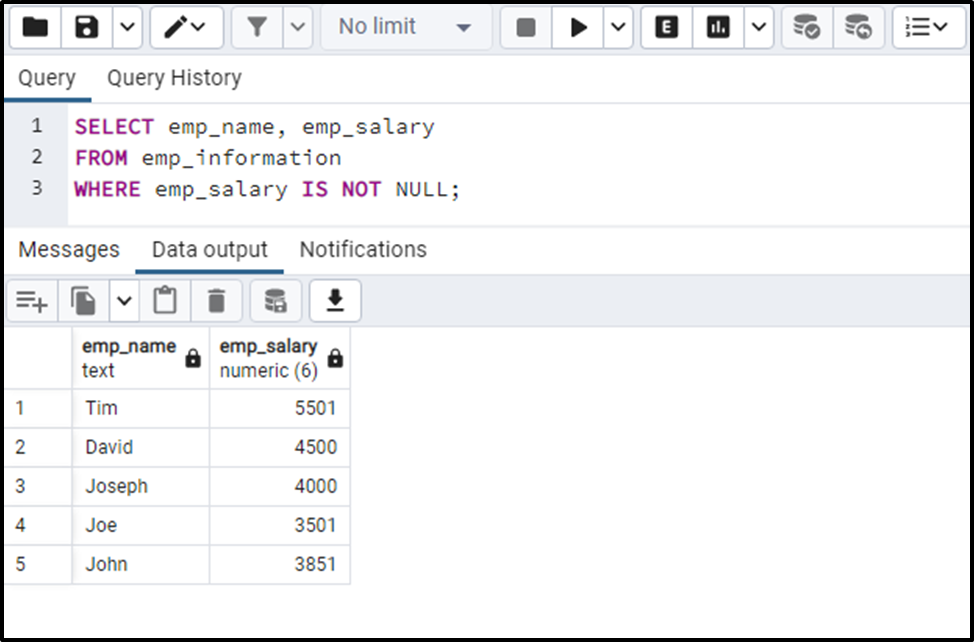Similarly, you can use the “IS NULL” operator, to find the null values in the specified column/expression.

Example 9: How to Use LIKE Operator in Postgres?

Use the LIKE operator to find the employees whose name starts with “J”:

```SELECT *
FROM emp_information
WHERE emp_name LIKE 'J%';```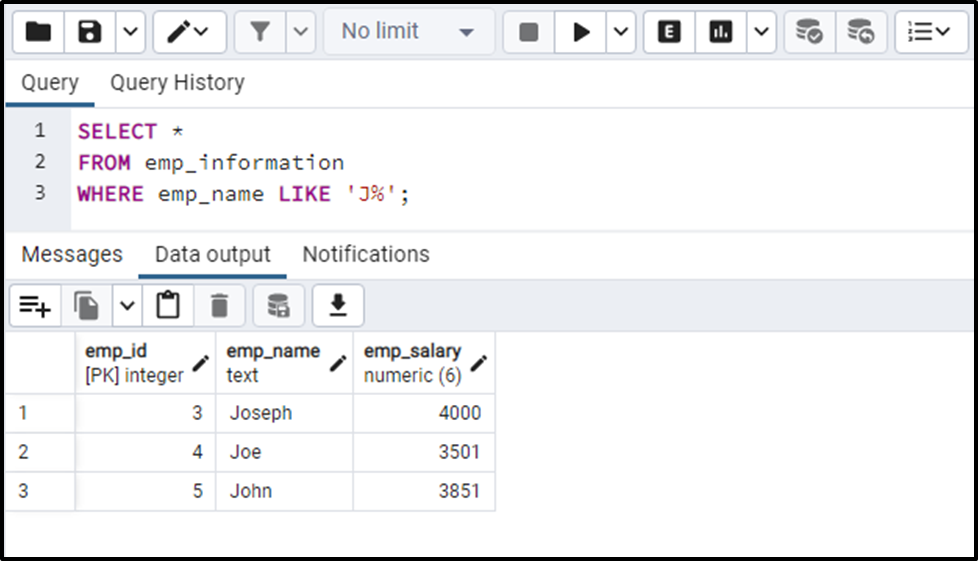The output shows that the LIKE operator retrieves the data of all those employees whose name starts with a letter “J”;

That’s all from this guide!

Conclusion

PostgreSQL offers a wide range of comparison operators, including basic and advanced ones, such as =, <, <>, BETWEEN, IN, etc. In Postgres, comparison operators are used for comparing values and determining whether they meet certain conditions/criteria. This blog explained the working of various comparison operators using practical examples.